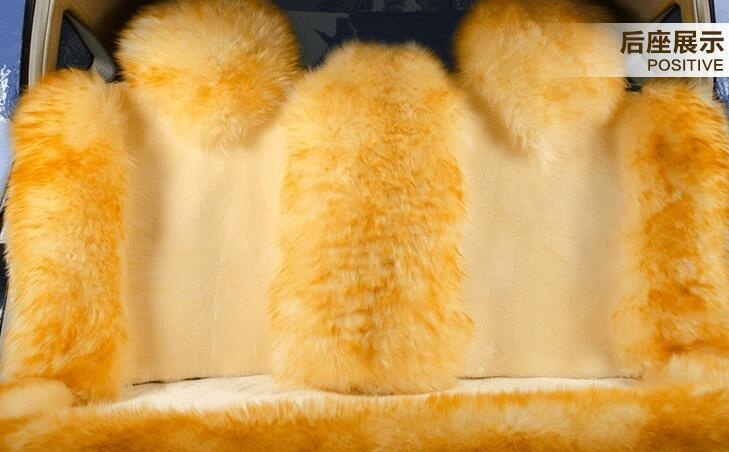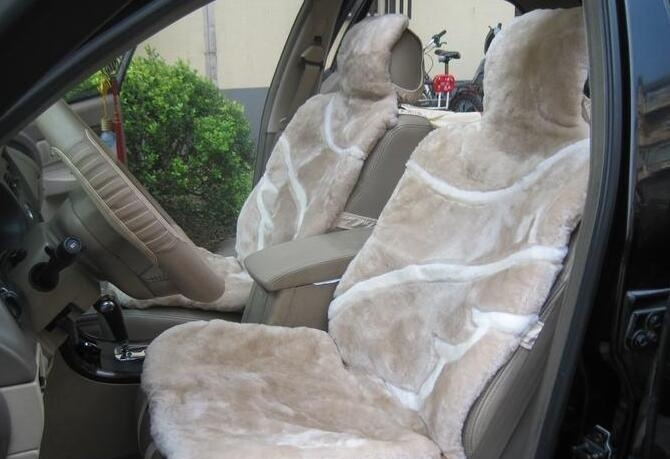|

# 汽车羊毛坐垫选购及保养技巧

羊毛汽车坐垫是冬季里大众选择之一，虽然很难打理，但也挡不住大家对它的热爱，随着社会科技不断发展市场上汽车羊毛坐垫已完全到了以假乱真的级别，用肉眼观察，简单试用都难以辨别真假，那汽车羊毛坐垫如何选购呢？下面就教一教大家汽车羊毛坐垫选购及保养技巧。如何选购汽车羊毛坐垫

汽车改装汪磊师傅介绍说，质量可靠的羊毛座垫采用优质羊皮经过鞣制、洗净、脱脂、去味、消毒、碳化、梳毛、剪烫、裁制等几十道生产工序精制而成。下面，汪师傅介绍了选购羊毛坐垫的4个方法。

方法1、需要仔细观察羊毛的颜色，看看是否正宗

一般优质羊毛坐垫采用的是国内外优质环保染料和助剂，毛色通常是鲜亮光泽、色彩也是柔和生动。而劣质货则色彩黯淡，容易掉色、串色。

方法2、用手轻轻抚摸羊毛的表面

优质羊毛坐垫的密度性好，手感细腻、有丝绒感；而劣质的汽车坐垫的手感粗糙、黏涩。用手分开毛查看是否存在皮毛裂缝、皮块拼接是否牢固、有无明显拼缝等。正常情况下优质的羊毛坐垫不轻易掉毛，更不会让碎毛沾在衣服上。

方法3、凑近去闻一闻，看羊毛坐垫是否有异味

优质羊毛坐垫在出厂前进行杀菌消毒，能防止螨虫、细菌滋生，且无任何异味；而劣质的羊毛由于油脂去除不净，会散发难闻的腥膻气味。

方法4、看汽车坐垫销量和好评率

如果车主准备网购羊毛坐垫，还有个最重要的数据我们应该好好分析考量一下，这就是“销量＋好评率”，这是一个市场检验好产品的核心竞争力强弱的最直接反映。汽车羊毛坐垫保养技巧

1、羊毛汽车坐垫，在使用的过程中均会有不同程度的毛还原卷曲现象。经常梳理或者用力抖动对保持本产品的特质和恢复其原有的自然形态，保持柔软、舒适很有帮助。

2、不用时请放在通风干燥的地方，勿重压。

3、任何采用澳大利亚进口绵羊毛皮制作的产品，在使用过程中均会出现不同程度的毛还原卷曲现象。在使用过一段时间后，请用梳子轻轻梳理皮毛即可。必要时可用电吹风吹梳，这样即可恢复其原有的自然形态，保持柔软、舒适，还可保持色泽的艳丽和图案的完整。不用时，请放在通风干燥的地方保存，勿使其折叠和重压。若使用防虫齐或樟脑丸时，应用纱布或卫生纸将其包好，勿使其直接接触毛皮。清理浮尘等，请在天气晴朗的进修进行，也可在室外用小木棒轻轻敲打皮毛的表面，以保持清洁卫生。必要时可使用家庭小型吸尘器吸尘清洁。需要晾晒时，请在通风处及半遮荫的条件下晒干或阴干。

4、若有轻微污染，请用清洁毛巾蘸稀释为1％浓度的专用清洗剂轻轻擦试污染处并用同样的方法用清水清洁，然后及时晾干。必要时可用梳子梳理，也可用电吹风轻轻吹干。

5、不得使产品接触高温（如开水、电热毯、阳光直晒等）及强性洗涤剂。若因包装或长期折叠造成产品表面羊毛倒状，请展开抖动数下，产品便会恢复原有弹性，这样不会损坏它的柔软品质。汽车羊毛坐垫清洗技巧

1、毡毛回松：坐垫使用后，毛与毛互相攀扯，形成毡块。用羊毛蓬松剂使其重新蓬松起来。

2、皮板防腐：皮板应定期防腐，避免因使用和存放不当而损坏。

3、清洗：祛除油污和灰尘。

4、回鲜：因氧化作用，有些毛面上看上去灰蒙蒙的，无论怎么洗也去不掉。必须用专配制剂处理，使之鲜艳。

5、复色：因磨损程度不同，褪色不匀。需复色使之色泽均匀。

6、拉直上光：使羊毛挺拔，整齐，光亮，柔滑。

7、整型：使坐垫平整。

十大汽车羊毛坐垫品牌

1、瑞丰

2、鑫博创

3、中澳

4、昊阳

5、澳世家

6、吉羊

7、澳客

8、澳都

9、大宋皮毛

10、香港上艺

`声明：本文由入驻焦点开放平台的作者撰写，除焦点官方账号外，观点仅代表作者本人，不代表焦点立场错误信息举报电话： 400-099-0099，邮箱：jubao@vip.sohu.com，或点此进行意见反馈，或点此进行举报投诉。`A B C D E F G H J K L M N P Q R S T W X Y Z
A - B - C - D - E
• A
• 鞍山
• 安庆
• 安阳
• 安顺
• 安康
• 澳门
• B
• 北京
• 保定
• 包头
• 巴彦淖尔
• 本溪
• 蚌埠
• 亳州
• 滨州
• 北海
• 百色
• 巴中
• 毕节
• 保山
• 宝鸡
• 白银
• 巴州
• C
• 承德
• 沧州
• 长治
• 赤峰
• 朝阳
• 长春
• 常州
• 滁州
• 池州
• 长沙
• 常德
• 郴州
• 潮州
• 崇左
• 重庆
• 成都
• 楚雄
• 昌都
• 慈溪
• 常熟
• D
• 大同
• 大连
• 丹东
• 大庆
• 东营
• 德州
• 东莞
• 德阳
• 达州
• 大理
• 德宏
• 定西
• 儋州
• 东平
• E
• 鄂尔多斯
• 鄂州
• 恩施
F - G - H - I - J
• F
• 抚顺
• 阜新
• 阜阳
• 福州
• 抚州
• 佛山
• 防城港
• G
• 赣州
• 广州
• 桂林
• 贵港
• 广元
• 广安
• 贵阳
• 固原
• H
• 邯郸
• 衡水
• 呼和浩特
• 呼伦贝尔
• 葫芦岛
• 哈尔滨
• 黑河
• 淮安
• 杭州
• 湖州
• 合肥
• 淮南
• 淮北
• 黄山
• 菏泽
• 鹤壁
• 黄石
• 黄冈
• 衡阳
• 怀化
• 惠州
• 河源
• 贺州
• 河池
• 海口
• 红河
• 汉中
• 海东
• 怀来
• I
• J
• 晋中
• 锦州
• 吉林
• 鸡西
• 佳木斯
• 嘉兴
• 金华
• 景德镇
• 九江
• 吉安
• 济南
• 济宁
• 焦作
• 荆门
• 荆州
• 江门
• 揭阳
• 金昌
• 酒泉
• 嘉峪关
K - L - M - N - P
• K
• 开封
• 昆明
• 昆山
• L
• 廊坊
• 临汾
• 辽阳
• 连云港
• 丽水
• 六安
• 龙岩
• 莱芜
• 临沂
• 聊城
• 洛阳
• 漯河
• 娄底
• 柳州
• 来宾
• 泸州
• 乐山
• 六盘水
• 丽江
• 临沧
• 拉萨
• 林芝
• 兰州
• 陇南
• M
• 牡丹江
• 马鞍山
• 茂名
• 梅州
• 绵阳
• 眉山
• N
• 南京
• 南通
• 宁波
• 南平
• 宁德
• 南昌
• 南阳
• 南宁
• 内江
• 南充
• P
• 盘锦
• 莆田
• 平顶山
• 濮阳
• 攀枝花
• 普洱
• 平凉
Q - R - S - T - W
• Q
• 秦皇岛
• 齐齐哈尔
• 衢州
• 泉州
• 青岛
• 清远
• 钦州
• 黔南
• 曲靖
• 庆阳
• R
• 日照
• 日喀则
• S
• 石家庄
• 沈阳
• 双鸭山
• 绥化
• 上海
• 苏州
• 宿迁
• 绍兴
• 宿州
• 三明
• 上饶
• 三门峡
• 商丘
• 十堰
• 随州
• 邵阳
• 韶关
• 深圳
• 汕头
• 汕尾
• 三亚
• 三沙
• 遂宁
• 山南
• 商洛
• 石嘴山
• T
• 天津
• 唐山
• 太原
• 通辽
• 铁岭
• 泰州
• 台州
• 铜陵
• 泰安
• 铜仁
• 铜川
• 天水
• 天门
• W
• 乌海
• 乌兰察布
• 无锡
• 温州
• 芜湖
• 潍坊
• 威海
• 武汉
• 梧州
• 渭南
• 武威
• 吴忠
• 乌鲁木齐
X - Y - Z
• X
• 邢台
• 徐州
• 宣城
• 厦门
• 新乡
• 许昌
• 信阳
• 襄阳
• 孝感
• 咸宁
• 湘潭
• 湘西
• 西双版纳
• 西安
• 咸阳
• 西宁
• 仙桃
• 西昌
• Y
• 运城
• 营口
• 盐城
• 扬州
• 鹰潭
• 宜春
• 烟台
• 宜昌
• 岳阳
• 益阳
• 永州
• 阳江
• 云浮
• 玉林
• 宜宾
• 雅安
• 玉溪
• 延安
• 榆林
• 银川
• Z
• 张家口
• 镇江
• 舟山
• 漳州
• 淄博
• 枣庄
• 郑州
• 周口
• 驻马店
• 株洲
• 张家界
• 珠海
• 湛江
• 肇庆
• 中山
• 自贡
• 资阳
• 遵义
• 昭通
• 张掖
• 中卫

1室1厅1厨1卫1阳台

1
2
3
4
5

0
1
2

1

1

0
1
2
3报名成功，资料已提交审核A B C D E F G H J K L M N P Q R S T W X Y Z
A - B - C - D - E
• A
• 鞍山
• 安庆
• 安阳
• 安顺
• 安康
• 澳门
• B
• 北京
• 保定
• 包头
• 巴彦淖尔
• 本溪
• 蚌埠
• 亳州
• 滨州
• 北海
• 百色
• 巴中
• 毕节
• 保山
• 宝鸡
• 白银
• 巴州
• C
• 承德
• 沧州
• 长治
• 赤峰
• 朝阳
• 长春
• 常州
• 滁州
• 池州
• 长沙
• 常德
• 郴州
• 潮州
• 崇左
• 重庆
• 成都
• 楚雄
• 昌都
• 慈溪
• 常熟
• D
• 大同
• 大连
• 丹东
• 大庆
• 东营
• 德州
• 东莞
• 德阳
• 达州
• 大理
• 德宏
• 定西
• 儋州
• 东平
• E
• 鄂尔多斯
• 鄂州
• 恩施
F - G - H - I - J
• F
• 抚顺
• 阜新
• 阜阳
• 福州
• 抚州
• 佛山
• 防城港
• G
• 赣州
• 广州
• 桂林
• 贵港
• 广元
• 广安
• 贵阳
• 固原
• H
• 邯郸
• 衡水
• 呼和浩特
• 呼伦贝尔
• 葫芦岛
• 哈尔滨
• 黑河
• 淮安
• 杭州
• 湖州
• 合肥
• 淮南
• 淮北
• 黄山
• 菏泽
• 鹤壁
• 黄石
• 黄冈
• 衡阳
• 怀化
• 惠州
• 河源
• 贺州
• 河池
• 海口
• 红河
• 汉中
• 海东
• 怀来
• I
• J
• 晋中
• 锦州
• 吉林
• 鸡西
• 佳木斯
• 嘉兴
• 金华
• 景德镇
• 九江
• 吉安
• 济南
• 济宁
• 焦作
• 荆门
• 荆州
• 江门
• 揭阳
• 金昌
• 酒泉
• 嘉峪关
K - L - M - N - P
• K
• 开封
• 昆明
• 昆山
• L
• 廊坊
• 临汾
• 辽阳
• 连云港
• 丽水
• 六安
• 龙岩
• 莱芜
• 临沂
• 聊城
• 洛阳
• 漯河
• 娄底
• 柳州
• 来宾
• 泸州
• 乐山
• 六盘水
• 丽江
• 临沧
• 拉萨
• 林芝
• 兰州
• 陇南
• M
• 牡丹江
• 马鞍山
• 茂名
• 梅州
• 绵阳
• 眉山
• N
• 南京
• 南通
• 宁波
• 南平
• 宁德
• 南昌
• 南阳
• 南宁
• 内江
• 南充
• P
• 盘锦
• 莆田
• 平顶山
• 濮阳
• 攀枝花
• 普洱
• 平凉
Q - R - S - T - W
• Q
• 秦皇岛
• 齐齐哈尔
• 衢州
• 泉州
• 青岛
• 清远
• 钦州
• 黔南
• 曲靖
• 庆阳
• R
• 日照
• 日喀则
• S
• 石家庄
• 沈阳
• 双鸭山
• 绥化
• 上海
• 苏州
• 宿迁
• 绍兴
• 宿州
• 三明
• 上饶
• 三门峡
• 商丘
• 十堰
• 随州
• 邵阳
• 韶关
• 深圳
• 汕头
• 汕尾
• 三亚
• 三沙
• 遂宁
• 山南
• 商洛
• 石嘴山
• T
• 天津
• 唐山
• 太原
• 通辽
• 铁岭
• 泰州
• 台州
• 铜陵
• 泰安
• 铜仁
• 铜川
• 天水
• 天门
• W
• 乌海
• 乌兰察布
• 无锡
• 温州
• 芜湖
• 潍坊
• 威海
• 武汉
• 梧州
• 渭南
• 武威
• 吴忠
• 乌鲁木齐
X - Y - Z
• X
• 邢台
• 徐州
• 宣城
• 厦门
• 新乡
• 许昌
• 信阳
• 襄阳
• 孝感
• 咸宁
• 湘潭
• 湘西
• 西双版纳
• 西安
• 咸阳
• 西宁
• 仙桃
• 西昌
• Y
• 运城
• 营口
• 盐城
• 扬州
• 鹰潭
• 宜春
• 烟台
• 宜昌
• 岳阳
• 益阳
• 永州
• 阳江
• 云浮
• 玉林
• 宜宾
• 雅安
• 玉溪
• 延安
• 榆林
• 银川
• Z
• 张家口
• 镇江
• 舟山
• 漳州
• 淄博
• 枣庄
• 郑州
• 周口
• 驻马店
• 株洲
• 张家界
• 珠海
• 湛江
• 肇庆
• 中山
• 自贡
• 资阳
• 遵义
• 昭通
• 张掖
• 中卫• 手机• 分享
• 设计
免费设计
• 计算器
装修计算器
• 入驻
合作入驻
• 联系
联系我们
• 置顶
返回顶部